cancel
Showing results for
Did you mean:

# Calculate Read Speed of HyperFlash – KBA220990Employee

## Calculate Read Speed of HyperFlash – KBA220990

Version: **

Translation - Japanese: ハイパー フラッシュのリード速度計算– KBA220990- Community Translated (JA)

Question:

What is the read speed of HyperFlash™ at 100 MHz? How do I calculate it?

Answer: See the HyperFlash datasheet (001-99198 Rev. *H or later) for all reference data used in the following calculations.

• Case 1: 4-byte random access read (e.g., pre-fetch a single instruction)
• Latency clock cycles are required according to Table 4 in the datasheet. A 10-clock latency is needed at 100 MHz
• As shown in datasheet Figure 31, it takes three clocks to send command-address, latency, and to read data out. This can be explained as follows:
• 1.5 clocks to send command-address (part of it) + 10 clocks latency at 100 MHz + 0.5 clock + 2 clocks to read data = total 14 clocks to read 2 words
• For back-to-back random reads, tCSHI and tCSS should be considered in the calculation. See datasheet Table 53:
• 10 ns (tCSHI) + 3 ns (tCSS) + (1.5 × 10 ns) + (10 × 10 ns) + (0.5 × 10 ns) + (2 × 10 ns) = 153 ns
• Read throughput is: 4 × 1000000000 / 153 = 26143790.85 bytes/s = 25 MB/s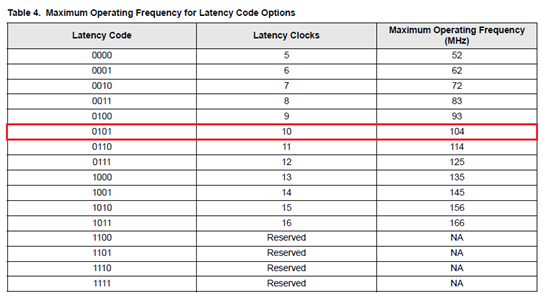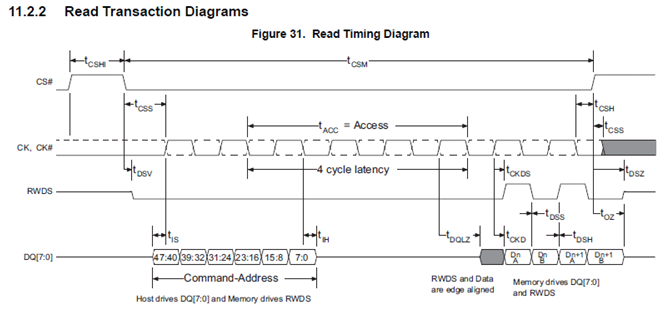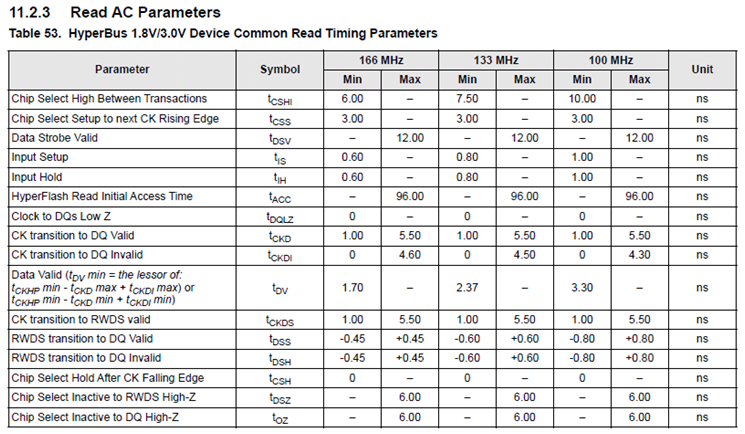• Case 2: Read transaction crossing a page boundary (e.g., reading an image from HyperFlash)
• Assume a host read address range of 0x0001 0000 to 0x0001 0FFF (read 4K words or 8K bytes)
• The initial latency is 10 clocks
• The initial page crossing latency is 0 clock (with 100 MHz, read starting address offset 0x0,1,2,3,4,5,6,8,9,A,B,C,D,E has no initial page crossing latency)
• 10 ns (tCSHI) + 3 ns (tCSS) + (1.5 × 10 ns) + (10 × 10 ns) + (0.5 × 10 ns) + (4096 word × 10ns) = 41093 ns
• Read throughput is: 8192 × 1000000000 / 41093 = 199352687 byte/s = 190.17 MB/s
• Assume a host read address range of 0x0001 0007 to 0x0001 1006 (read 4K words or 8K bytes)
• The initial latency is 10 clocks
• The initial page crossing latency is 1 clock (with 100 MHz, read starting address offset 0x7,F has one initial page crossing latency)
• 10 ns (tCSHI) + 3 ns (tCSS) + (1.5 × 10 ns) + (10 × 10 ns) + (0.5 × 10 ns) + (9-word × 10 ns) + (1 initial page crossing latency × 10 ns) + ((4096 - 9) word × 10 ns) = 41103 ns
• Read throughput is: 8192 × 1000000000 / 41103 = 199304187 byte/s = 190.07 MB/s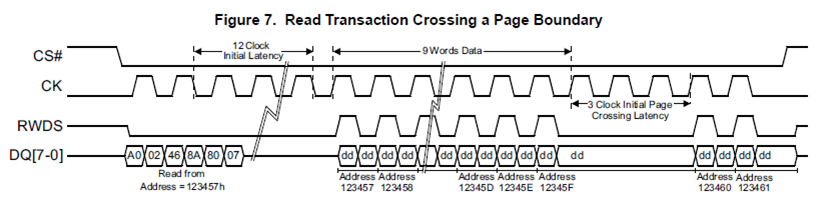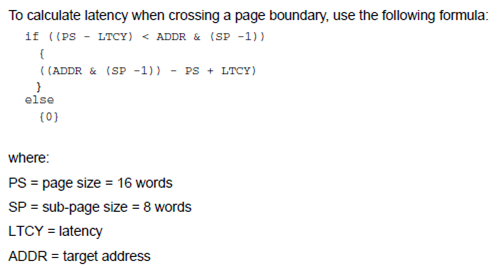Version history
Revision #:
1 of 1
Last update:
‎Sep 25, 2017 12:22 AM
Updated by:
Contributors